Function Repository Resource:

# TransitiveGraphQ

Test whether the binary relation defined by edges of a graph is transitive

Contributed by: Wolfram Staff (original content by Sriram V. Pemmaraju and Steven S. Skiena)
 ResourceFunction["TransitiveGraphQ"][g] yields True if the graph g is transitive and False otherwise.

## Details and Options

ResourceFunction["TransitiveGraphQ"][g] returns True if the binary relation defined by edges of the graph g is transitive.

## Examples

### Basic Examples (2)

A directed graph formed by tuples is transitive:

 In:=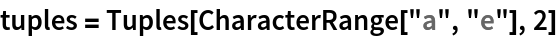Out=In:=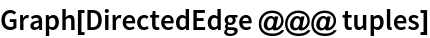Out=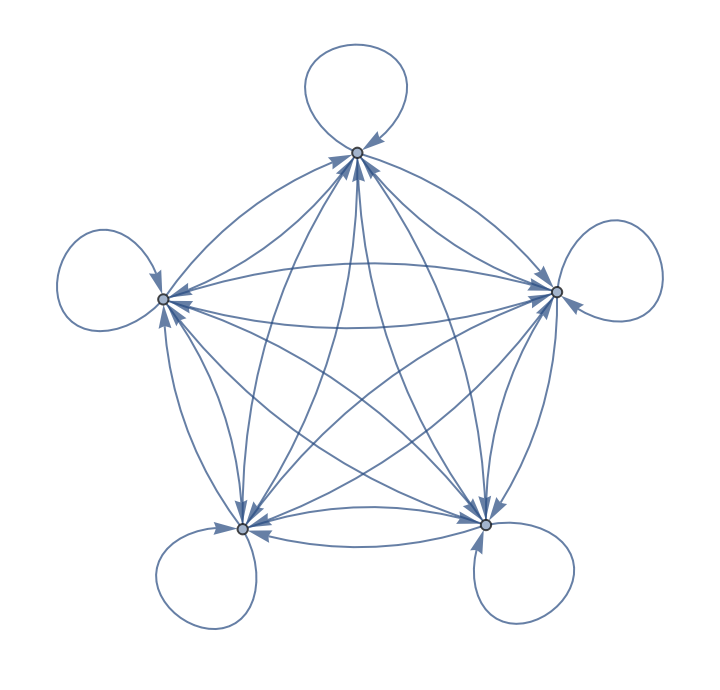In:=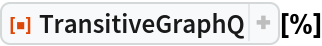Out=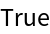But the undirected graph formed by the same tuples is not:

 In:=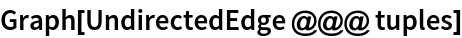Out=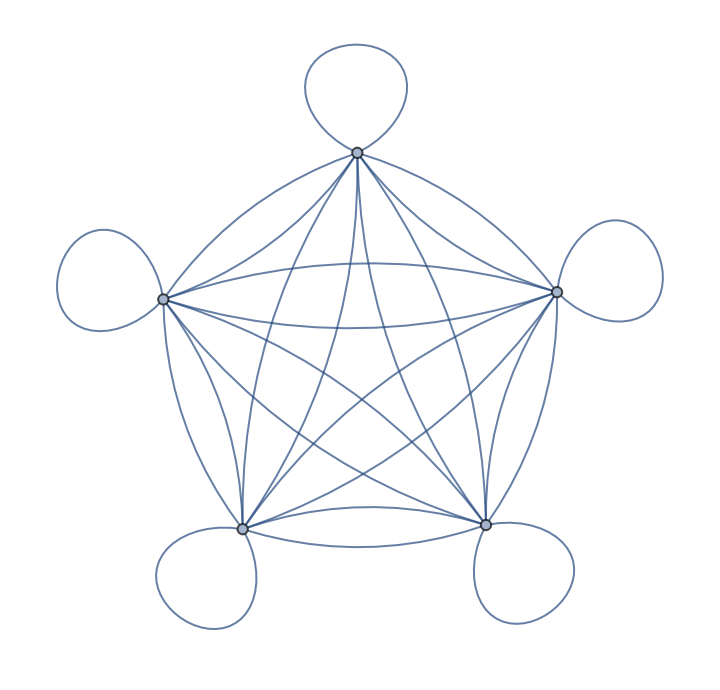In:=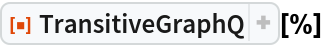Out=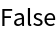The divisibility relation between integers is transitive since n|m implies m=kn for some integer k, so m|l implies n|l:

 In:=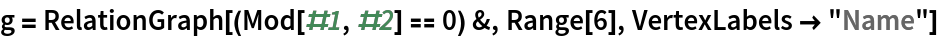Out=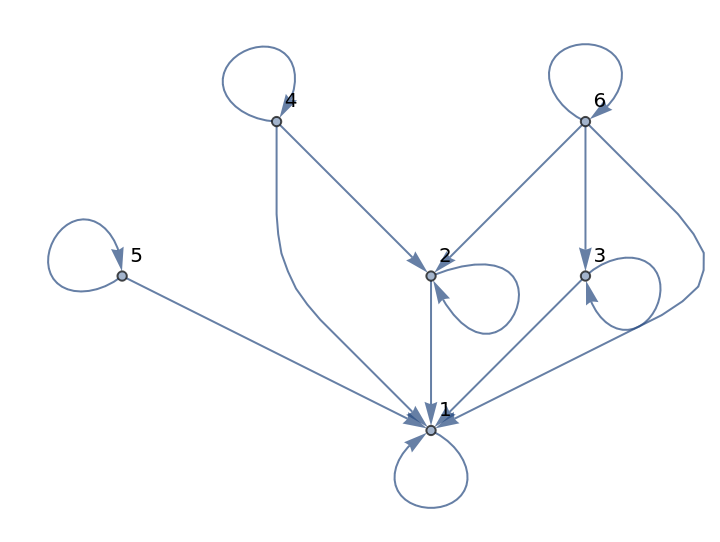In:=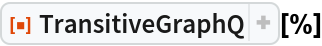Out=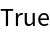## Version History

• 1.0.0 – 01 July 2020